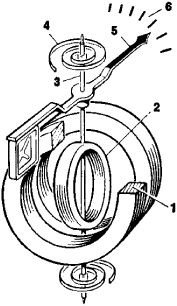# Electrodynamic Instrument

## electrodynamic instrument

[i‚lek·trō·dī′nam·ik ′in·strə·mənt]
(engineering)
An instrument that depends for its operation on the reaction between the current in one or more movable coils and the current in one or more fixed coils. Also known as electrodynamometer.

## Electrodynamic Instrument

a measuring instrument whose operation is based on the mechanical interaction between two conductors in which an electric current is present. It consists of a measuring transducer, which converts the quantity being measured into an alternating or direct current, and a measuring mechanism of an electrodynamic system (Figure 1). The most common electrodynamic instruments have a fixed coil, inside of which is a movable coil and a pointer on a spindle. A torque is developed around the spindle by the interaction of the currents in the two coils and is proportional to the product of the effective values of the currents. A countertorque is created by a spring connected to the spindle. The pointer comes to rest when the two torques are equal.Figure 1. Electrodynamic measuring instrument: (1) fixed coil, (2) movable coil, (3) spindle, (4) spring, (5) pointer, (6) scale

Electrodynamic instruments are the most accurate electrical measuring instruments used to determine the effective values of currents and voltages in AC and DC circuits. When the windings of the coils are connected in series, the angle of rotation of the pointer is proportional to the square of the quantity being measured. Series connection of the windings is used for voltage and current measurements (voltmeters and ammeters). Electrodynamic measuring mechanisms are also used for power measurements (wattmeters). In this case a current proportional to the current in the circuit being measured is passed through the fixed coil, and a current proportional to the voltage in the circuit being measured is passed through the movable coil. The instrument’s readings are proportional to the active or reactive electric power. Electrodynamic mechanisms in the form of ratio meters may also be used as frequency meters, phase meters, and faradmeters.

Electrodynamic instruments are manufactured chiefly as portable, highly accurate instruments in three classes of precision: 0.1, 0.2, and 0.5. There is also a ferrodynamic type in which a magnetic circuit made of a ferromagnetic material is used to increase the strength of the magnetic field of the fixed coil. Such instruments are designed to withstand vibration, shaking, and shocks; their classes of precision are 1.5 and 2.5.

### REFERENCE

Elektricheskie izmereniia. Edited by E. G. Shramkov. Moscow, 1972.

N. N. VOSTROKNUTOV

Site: Follow: Share:
Open / Close